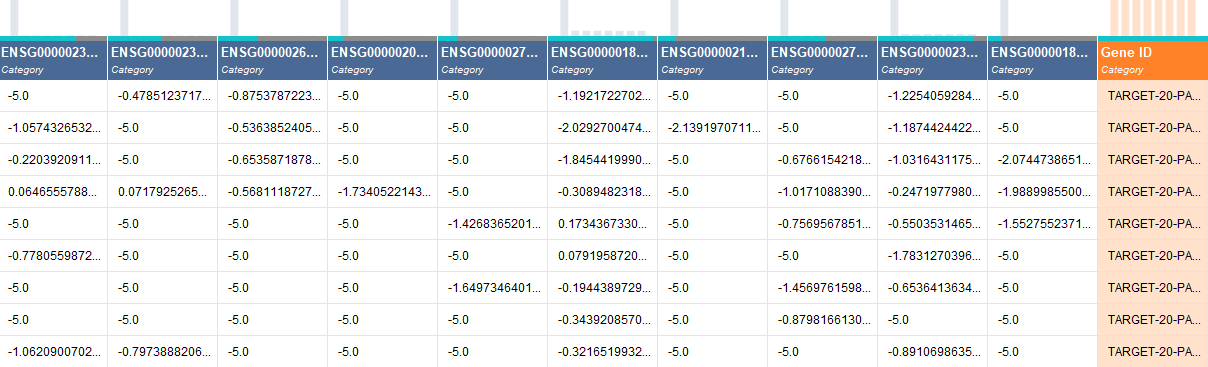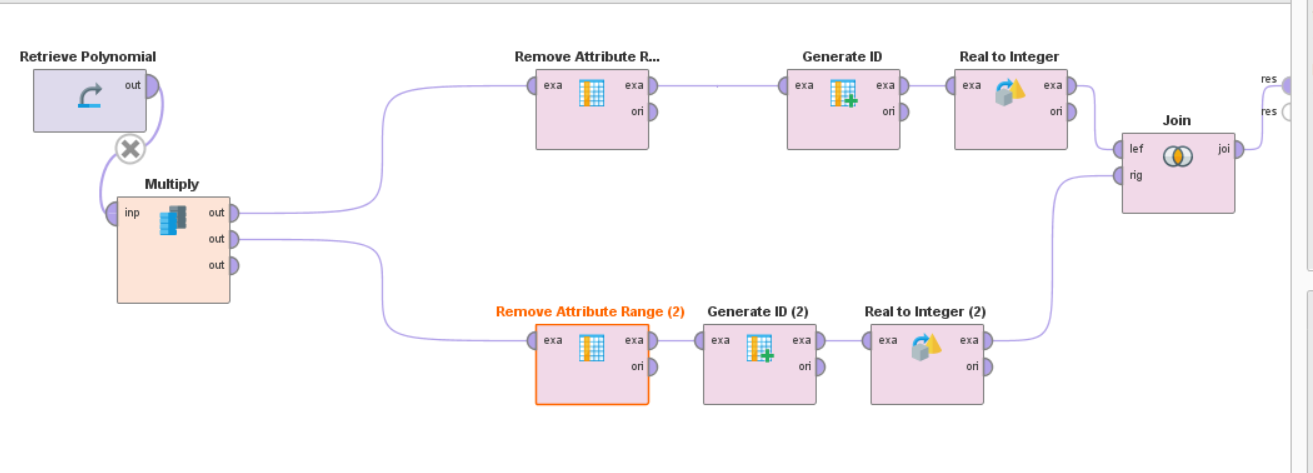# Change types of attributes for a large amount of data

Member Posts: 9Contributor I
Hi,

I have a set of genetics data with more that 30,000 attributes. All of these attributes are in the categorical(nominal) data type, but I want to change all of them except for one to numerical data type . I couldn't do it by adding the operator "Nominal to Numerical" in the data flow because apparently RapidMiner Studio only supports up to 16384 attributes.

So I'm trying to change the data types from Turbo Prep, but I don't want to manually change the type for each attribute one by one. I'm wondering if there's a way to select all/multiple attributes in Turbo Prep and change everything at once?

Or if there are any other ways?

I attached a screenshot of a sample of my data. Hopefully that helps!Thanks!

• Moderator, Member Posts: 1,207Unicorn
edited July 2019 Solution Accepted
Hello @gracewei

I never knew that there was an attribute limitation in RM. Based on your question, I came up with two solutions, one through turbo prep and another through the process window with nominal to numerical.

1. In turbo prep, you can select all the columns by pressing "ALT" key on the keyboard and click on the first attribute with category type, it will select all the attributes of category type. Then you can go to change the type and change them to number.

2. I got one idea with nominal to numerical operator and there may be other simple ways as well but you can try this before someone responds with a much easier way. I tried something like splitting the dataset into two parts, as your data has 30000 attributes you can split 15000 each. Then generate ID for both the datasets, this ID will help for joining these attributes back into 30000 dataset. After this, for each 15000 attribute dataset, we apply the nominal to the numerical operator and then join them back with "id" as primary key using join operator.

I tried this with the polynomial dataset in the samples, below I attached XML process for your understanding, you can copy this XML code into an XML window or RM and click on green tick mark you can see the process. I also provided the process image below with explanation.

<?xml version="1.0" encoding="UTF-8"?><process version="9.3.001">
<context>
<input/>
<output/>
<macros/>
</context>
<operator activated="true" class="process" compatibility="9.3.001" expanded="true" name="Process">
<parameter key="logverbosity" value="init"/>
<parameter key="random_seed" value="2001"/>
<parameter key="send_mail" value="never"/>
<parameter key="process_duration_for_mail" value="30"/>
<parameter key="encoding" value="SYSTEM"/>
<process expanded="true">
<operator activated="true" class="retrieve" compatibility="9.3.001" expanded="true" height="68" name="Retrieve Polynomial" width="90" x="179" y="34">
<parameter key="repository_entry" value="//Samples/data/Polynomial"/>
</operator>
<operator activated="true" class="multiply" compatibility="9.3.001" expanded="true" height="124" name="Multiply" width="90" x="313" y="34"/>
<operator activated="true" class="remove_attribute_range" compatibility="9.3.001" expanded="true" height="82" name="Remove Attribute Range (2)" width="90" x="581" y="136">
<parameter key="first_attribute" value="4"/>
<parameter key="last_attribute" value="5"/>
</operator>
<operator activated="true" class="generate_id" compatibility="9.3.001" expanded="true" height="82" name="Generate ID (2)" width="90" x="744" y="136">
<parameter key="create_nominal_ids" value="false"/>
<parameter key="offset" value="0"/>
</operator>
<operator activated="true" class="real_to_integer" compatibility="9.3.001" expanded="true" height="82" name="Real to Integer (2)" width="90" x="878" y="136">
<parameter key="attribute_filter_type" value="all"/>
<parameter key="attribute" value=""/>
<parameter key="attributes" value=""/>
<parameter key="use_except_expression" value="false"/>
<parameter key="value_type" value="real"/>
<parameter key="use_value_type_exception" value="false"/>
<parameter key="except_value_type" value="real"/>
<parameter key="block_type" value="value_series_end"/>
<parameter key="use_block_type_exception" value="false"/>
<parameter key="except_block_type" value="value_series_end"/>
<parameter key="invert_selection" value="false"/>
<parameter key="include_special_attributes" value="false"/>
<parameter key="round_values" value="false"/>
</operator>
<operator activated="true" class="remove_attribute_range" compatibility="9.3.001" expanded="true" height="82" name="Remove Attribute Range" width="90" x="447" y="34">
<parameter key="first_attribute" value="1"/>
<parameter key="last_attribute" value="3"/>
</operator>
<operator activated="true" class="generate_id" compatibility="9.3.001" expanded="true" height="82" name="Generate ID" width="90" x="581" y="34">
<parameter key="create_nominal_ids" value="false"/>
<parameter key="offset" value="0"/>
</operator>
<operator activated="true" class="real_to_integer" compatibility="9.3.001" expanded="true" height="82" name="Real to Integer" width="90" x="782" y="34">
<parameter key="attribute_filter_type" value="all"/>
<parameter key="attribute" value=""/>
<parameter key="attributes" value=""/>
<parameter key="use_except_expression" value="false"/>
<parameter key="value_type" value="real"/>
<parameter key="use_value_type_exception" value="false"/>
<parameter key="except_value_type" value="real"/>
<parameter key="block_type" value="value_series_end"/>
<parameter key="use_block_type_exception" value="false"/>
<parameter key="except_block_type" value="value_series_end"/>
<parameter key="invert_selection" value="false"/>
<parameter key="include_special_attributes" value="false"/>
<parameter key="round_values" value="false"/>
</operator>
<operator activated="true" class="concurrency:join" compatibility="9.3.001" expanded="true" height="82" name="Join" width="90" x="1050" y="85">
<parameter key="remove_double_attributes" value="true"/>
<parameter key="join_type" value="inner"/>
<parameter key="use_id_attribute_as_key" value="false"/>
<list key="key_attributes">
<parameter key="id" value="id"/>
</list>
<parameter key="keep_both_join_attributes" value="false"/>
</operator>
<connect from_op="Retrieve Polynomial" from_port="output" to_op="Multiply" to_port="input"/>
<connect from_op="Multiply" from_port="output 1" to_op="Remove Attribute Range" to_port="example set input"/>
<connect from_op="Multiply" from_port="output 2" to_op="Remove Attribute Range (2)" to_port="example set input"/>
<connect from_op="Multiply" from_port="output 3" to_port="result 1"/>
<connect from_op="Remove Attribute Range (2)" from_port="example set output" to_op="Generate ID (2)" to_port="example set input"/>
<connect from_op="Generate ID (2)" from_port="example set output" to_op="Real to Integer (2)" to_port="example set input"/>
<connect from_op="Real to Integer (2)" from_port="example set output" to_op="Join" to_port="right"/>
<connect from_op="Remove Attribute Range" from_port="example set output" to_op="Generate ID" to_port="example set input"/>
<connect from_op="Generate ID" from_port="example set output" to_op="Real to Integer" to_port="example set input"/>
<connect from_op="Real to Integer" from_port="example set output" to_op="Join" to_port="left"/>
<connect from_op="Join" from_port="join" to_port="result 2"/>
<portSpacing port="source_input 1" spacing="0"/>
<portSpacing port="sink_result 1" spacing="0"/>
<portSpacing port="sink_result 2" spacing="0"/>
<portSpacing port="sink_result 3" spacing="0"/>
</process>
</operator>
</process>

Process image below:
In the below image, you can see I multiply the dataset so that I can separate attributes. Then I connected it to "Remove attribute Range", here as you have 30000 attributes, the input of the first one is 1 to 15000 and the second one is 15001 to 30000. Be careful while giving these numbers as the first and last attribute are removed as well, do it similarly. Then, I "generate ID" for both of them and converted the attributes from real to integer (based on the sample data), you can use nominal to numerical, then join them based on ID column.Hope this helps, as I tried this on 5 attribute dataset it worked, try with your dataset and inform if it helps.

Regards,
Varun
https://www.varunmandalapu.com/

# Be Safe. Follow precautions and Maintain Social Distancing

• Member Posts: 9Contributor I
Wow! @varunm1 Thank you so so much for such detailed response! You're a life saver!

I already tried your first method, and it worked perfectly! I will also try the second approach.

• Administrator, Employee, RapidMiner Certified Analyst, RapidMiner Certified Expert, RMResearcher, Member Posts: 164RM Research
Hi @gracewei,

So there can't be a hard limit on the number of attributes in RM (at least not at ~16000). I just created an ExampleSet with 1000000 attributes (and 10 examples) with the Generate Data operator.

I think the problem here is the Nominal to Numerical operator. This operator is not parsing numbers, but performs a coding of the categorical types. So for example if you would have a color attribute with red, yellow, green as values, you would get 3 attributes, "color = red", "color = yellow", "color = green".

When I see this correctly you basically have numerical attributes who are just read in as categorical. So Nominal to Numerical operator would create for every value in every categorical attribute, a new attribute. As probably you have a bunch of different values in the attributes you would end up with an enormous number of attributes.

Have a look at the Parse Numbers operators, which probably do what you want to achieve.

Best regards,
Fabian

PS.: Maybe interesting for you as well @varunm1 , With the Merge Attributes operator from the operator toolbox extension you can merge ExampleSets together (like joining row by row) without the need of an ID or the overhead of the Join operator.

• Moderator, Member Posts: 1,207Unicorn

Thanks Fabian, I will check it out.

Regards,
Varun
https://www.varunmandalapu.com/

# Be Safe. Follow precautions and Maintain Social Distancing

• Member Posts: 9Contributor I
@tftemme I see. Thank you so much for the explanation and advice!
• Member Posts: 1Newbie
1. In Master Data Manager, click System Administration.

2. On the Manage Model page, select a model from the grid and then click Entities.

3. On the Manage Entity page, select the row for the entity that you want to create an attribute for.

4. Click Attributes.

5. If the attribute is for leaf members, select Leaf from the Member Types list box.

6. If the attribute is for consolidated members, select Consolidated from the Member Types list box.